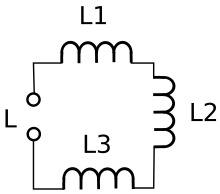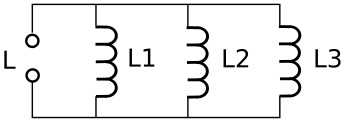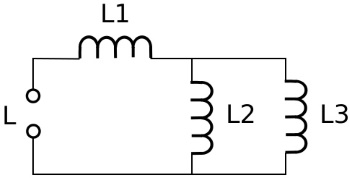#Inductors in Series and Parallel by Stephen Bucaro

The Unit of Inductance

When a power source is applied across a coil, current flow is low at first because a magnetic field starts to build in the coil and the changing magnetic field causes back electromotive force (EMF) which opposes the flow of current. As the current builds, the rate of increase of current slows, and therefore the rate of change of the magnetic field decreases. The rate at which current changes depends upon the inductance of the coil.

Inductance is measured in a unit called a henry (H). If the rate of current change through an inductor is one ampere per second and that causes 1 volt of EMF across an inductor, than that inductor has an inductance of 1 H. The henry is a very large unit of inductance. inductances are usually expressed in millihenrys (mH), micro#henrys (uH), or even nanohenrys (nH).

1 mH = 0.001 H
1 uH = 0.001 mH
1 nH = 0.001 uH

The amount of inductance in a coil generally depends upon the number of turns and the material used for the core. The more turns the higher the inductance. Having a ferromagnetic core increases the inductance.

Inductors in SeriesWith inductors in series, the total inductance is the sum of the inductances of the individual inductors, You just add up the values. In the example shown above, if L1 = 30 uH, L2 = 40 uH, and L3 - 50 uH, then L total = 30 + 40 + 50 = 120 uH.

Inductors in ParallelWith inductors in parallel, the reciprocal of the equivalent inductance is the sum of the reciprocals of the inductors in parallel. In the example shown above, if L1 = 30 uH, L2 = 40 uH, and L3 - 50 uH, then 1/L equivalent = 1/30 + 1/40 + 1/50 = 12.8 uH.

Inductors in Series and ParallelWith inductors that are connected in series and parallel, calculate the equivalent inductance of the parallel path, and then use the value as if it were a single inductor in series.

In the example above, if L1 = 30 uH, L2 = 40 uH, and L3 - 50 uH, then the equivalent inductance of L2 and L3 in parallel is 1/L equivalent = 1/40 + 1/50 = 22 uH. Then the total inductance is 30uH + 22uH = 52 uH.

In these calculations, don't mix units. Don't mix millihenrys with microhenrys, or microhenrys with nanohenrys. The units used for the individual components will be the units to use for the final answer.

These calculations assume that there is no interactions of the the magnetic fields around inductors. If there is mutual inductance among series or parallel connected inductors, then you have a much more complicated calculation.